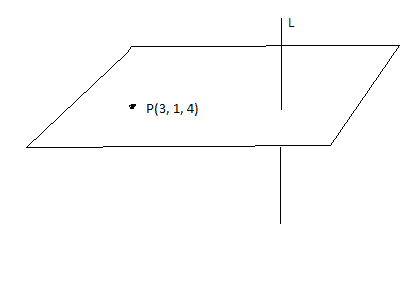# Find a point on the line closest to another point

• MarcL
The distance of P from the normal line is the length of the line drawn perpendicularly to the normal...where is that line?The normal, but... it seems kinda redundant if it lies on the same line , anyway to me at least.The normal, but... it seems kinda redundant if it lies on the same line , anyway to me at least.Of course, it intersects the normal, but what is the position of the line drawn from P and perpendicular to the normal, with respect to the plane? Try to draw a picture.f

## Homework Statement

(2 part problem) a) A plane passes through the point P(3,1,4) and is orthogona to the line (x-1)/2 = (2-y)/-7 = z-3
b) Find the point on the line closest to point (3,1,4)

## Homework Equations

Symmetric equation --> (x-x1)/t = (y-y1)/t = (z-z1)/t ( anyway i think that's what it is

## The Attempt at a Solution

I found the equation of the plane using the direction vector as (2,-7,1)
and used this form 2(x-3)-7(y-1)+(z-4)= 0

I can't seem to be able to go on to start b. I would think of plugging P in but that seems way too easy.

Any idea on how I can approach this?

I found the equation of the plane using the direction vector as (2,-7,1)
and used this form 2(x-3)-7(y-1)+(z-4)= 0

I can't seem to be able to go on to start b. I would think of plugging P in but that seems way too easy.

Any idea on how I can approach this?
Where will that closest point be in relation to the plane?

## The Attempt at a Solution

I found the equation of the plane using the direction vector as (2,-7,1)
Check the sign of the y component of the direction vector.

and used this form 2(x-3)-7(y-1)+(z-4)= 0
I can't seem to be able to go on to start b. I would think of plugging P in but that seems way too easy.

Any idea on how I can approach this?

The distance of P from the normal line is the length of the line drawn perpendicularly to the normal...Where is that line?

The normal, but... it seems kinda redundant if it lies on the same line , anyway to me at least.

The normal, but... it seems kinda redundant if it lies on the same line , anyway to me at least.
Of course, it intersects the normal, but what is the position of the line drawn from P and perpendicular to the normal, with respect to the plane? Try to draw a picture.

If you can not see it, write the distance of any point of the normal line from point P. When is it minimum, and what is that minimum distance?

Drawing a picture, as ehild suggested, is an excellent idea. Having an image to look at gives you insights that formulas and equations simply can't provide. This took me a couple of minutes to draw.